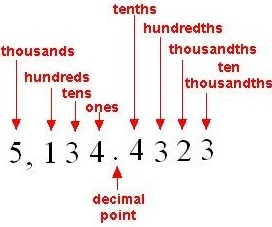# Decimal Place Value: Tenths and Hundredths

#### Complete Python Prime Pack

9 Courses     2 eBooks

#### Artificial Intelligence & Machine Learning Prime Pack

6 Courses     1 eBooks

#### Java Prime Pack

9 Courses     2 eBooks

The value of a digit based on its position or place in a number is known as its place value.

The following are the place values to the left of decimal point: the ones, the tens, the hundreds, the thousands and so on.

The following are the place values to the right of decimal point: the tenth, the hundredth, the thousandth, the ten thousandth and so on.

The place values are divided into groups of 3 digits each. The first group called the ones group consists of the ones place, tens place and the hundreds place. The thousands group consists of the thousands place, ten thousands place and the hundred thousands place. The millions group consists of the millions place, ten millions’ place and the hundred millions place. The groups of 3 digits are separated by commas.

For example: Consider the number 5,134.4323. The place value names of different digits in the number are shown in the figure below.Give the digits in ones place and tenths place in the number 2.104

### Solution

Step 1:

In the given number 2.104, the digit in ones place is 2 as it is the first digit to the left of decimal point.

Step 2:

The digit in tenths place is 1 as it is the first digit to the right of decimal point

Give the digits in tens place and hundredths place in the number 52.761

### Solution

Step 1:

In the given number 52.761, the digit in tens place is 5 as it is the second digit to the left of decimal point.

Step 2:

The digit in hundredths place is 6 as it is the second digit to the right of decimal point.

Give the digits in tens place and tenths place in the number 34.59

### Solution

Step 1:

In the given number 34.59, the digit in tens place is 3 as it is the second digit to the left of decimal point.

Step 2:

The digit in tenths place is 5 as it is the first digit to the right of decimal point.